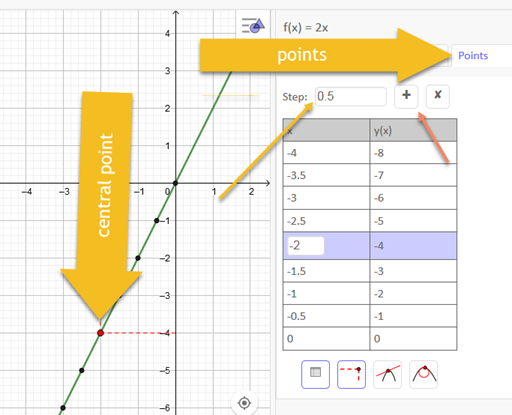# Is there a way to automatically to get the coordinates of a polynomial?

Justinj001 shared this question 5 years ago

Is it possible to automatically get the coordinates of a polynomial by the unit shown in the grid. For example if the x coordinates are distanced by 1 unit, then the coordinates for each x i.e for -3,-2,-1,0,1,2,3 should be obtained. I'm right now manually selecting the points on the curve to get the coordinates of the polynomial drawn by GeoGebra.1

f(x) polynomial or function

sequence((k,f(k)),k,-5,5,1) (you can change the numbers)1

you can use function inspector also1

I couldn't get this answer. Could you illustrate the answer with a .ggb file.1

sequence

function inspector under angle icon in popup menus

Files: foro.ggb1

Sorry for the late reply. That definitely brought the result I want. Great job. Please tell me how to use the function inspector. I couldn't get how to automatically get the points using function inspector.11

I tried plotting f(x)=2x, and using the function inspector I got only two points A and B. Could you tell how to get the other coordinates of the function f(x)=2x. Although it shows two points in the input tab, I could only see one point(i.e B) in the graph.11